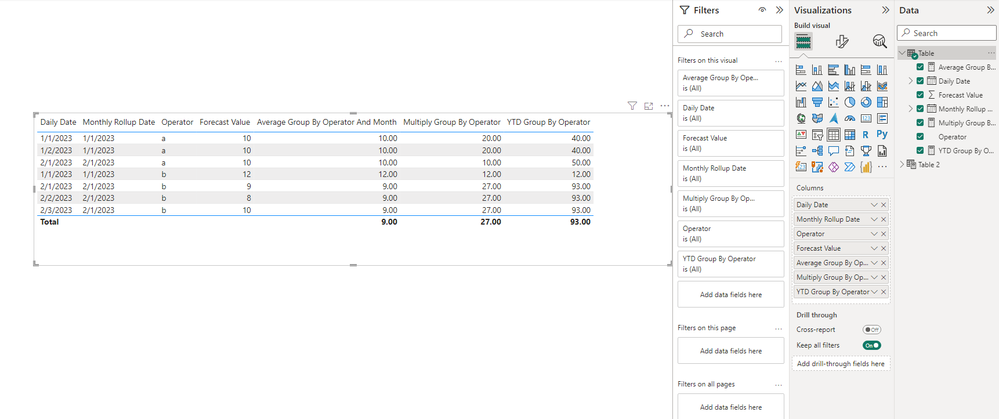cancel
Showing results for
Did you mean:

Fabric is Generally Available. Browse Fabric Presentations. Work towards your Fabric certification with the Cloud Skills Challenge.Frequent Visitor

## Calculate Daily Average per Month, Sum Results YTD

Hi all,

I'm struggling with a clean way to calculate the daily average per month and then sum those values YTD. To better describe what I'm after.. Here is my table setup:

 Daily Date Monthly Rollup Date Operator Forecast Value 1/1/2023 1/1/2023 a 10 1/1/2023 1/1/2023 b 12 1/2/2023 1/1/2023 a 10 ... ... ... 2/1/2023 2/1/2023 a 15

So the goal would be:

1. Calculate the average daily forecast per day across all operators by month
2. Multiply that daily average by how many days are in that given month
3. Sum each monthly total to get YTD

Let me know if this isn't detailed enough and I can add additional detail. I can see how to do this by creating a ton of measures for each month, however I am trying not to create 20 or so measures to accomplish this.

1 ACCEPTED SOLUTIONCommunity Support

Hi @fetterr ,

You can create the following measures:

``Average Group By Operator And Month = CALCULATE(AVERAGE('Table'[Forecast Value]),FILTER(ALLSELECTED('Table'),[Operator]=MAX('Table'[Operator])&&[Monthly Rollup Date]=MAX('Table'[Monthly Rollup Date])))``
``````Multiply Group By Operator =
var _day=COUNTROWS(FILTER(ALLSELECTED('Table'),[Operator]=MAX('Table'[Operator])&&[Monthly Rollup Date]=MAX('Table'[Monthly Rollup Date])))
return
_day*[Average Group By Operator And Month]``````
``YTD Group By Operator = SUMX(FILTER(ALLSELECTED('Table'),[Operator]=MAX('Table'[Operator])&&[Monthly Rollup Date]<=MAX('Table'[Monthly Rollup Date])),[Multiply Group By Operator])``The calculations are grouped according to the Operator, if you don't want to group, just remove that part.

Best Regards,

Stephen Tao

If this post helps, then please consider Accept it as the solution to help the other members find it more quickly.Community Support

Hi @fetterr ,

You can create the following measures:

``Average Group By Operator And Month = CALCULATE(AVERAGE('Table'[Forecast Value]),FILTER(ALLSELECTED('Table'),[Operator]=MAX('Table'[Operator])&&[Monthly Rollup Date]=MAX('Table'[Monthly Rollup Date])))``
``````Multiply Group By Operator =
var _day=COUNTROWS(FILTER(ALLSELECTED('Table'),[Operator]=MAX('Table'[Operator])&&[Monthly Rollup Date]=MAX('Table'[Monthly Rollup Date])))
return
_day*[Average Group By Operator And Month]``````
``YTD Group By Operator = SUMX(FILTER(ALLSELECTED('Table'),[Operator]=MAX('Table'[Operator])&&[Monthly Rollup Date]<=MAX('Table'[Monthly Rollup Date])),[Multiply Group By Operator])``The calculations are grouped according to the Operator, if you don't want to group, just remove that part.

Best Regards,

Stephen Tao

If this post helps, then please consider Accept it as the solution to help the other members find it more quickly.Announcements#### Power BI Monthly Update - November 2023

Check out the November 2023 Power BI update to learn about new features.#### Fabric Community News unified experience

Read the latest Fabric Community announcements, including updates on Power BI, Synapse, Data Factory and Data Activator.#### The largest Power BI and Fabric virtual conference

130+ sessions, 130+ speakers, Product managers, MVPs, and experts. All about Power BI and Fabric. Attend online or watch the recordings.Top Solution Authors
Top Kudoed Authors
Users online (3,999)